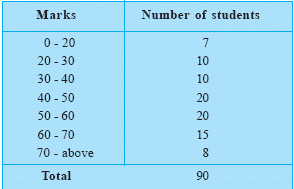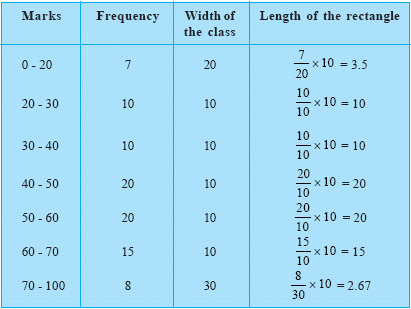Email us to get an instant 20% discount on highly effective K-12 Math & English kwizNET Programs!

#### Online Quiz (WorksheetABCD)

Questions Per Quiz = 2 4 6 8 10

### Middle/High School Algebra, Geometry, and Statistics (AGS)9.15 Histogram

 Data in the tabular form is as follows Histogram representing the dataIf we observe the graph carefully, we realize that the data has been misinterpreted.
As we know areas of the rectangle should be proportional to the frequencies in a histogram. Now look at the chart below.If we go by the rules we realize that there is an ! ERROR ! in the first and the last class interval.
We take the following steps to rectify the error
1.We select an approriate class size. The best suitable would be 10.
2.The lengths of the rectangle are then modified to be proportionate to the class size.

 After the ammendments the table would look like The corresponding histogram would then look likeDirections: On the basis of the given information answer the following questions

 Q 1: The width of the rectangle is same when they have the same class size.truefalse Q 2: If the class size of any class interval is different from the other then the length of the rectangle should be modified to make it proportionate to the class sizeFalseTrue Q 3: Which two class intervals have the same highest frequency?0 - 20 and 70 - 10030 - 40 and 50 - 6040 - 50 and 50 - 6040 - 50 and 60 - 70 Q 4: How many students didn't score more than 50% marks43476050 Question 5: This question is available to subscribers only! Question 6: This question is available to subscribers only!